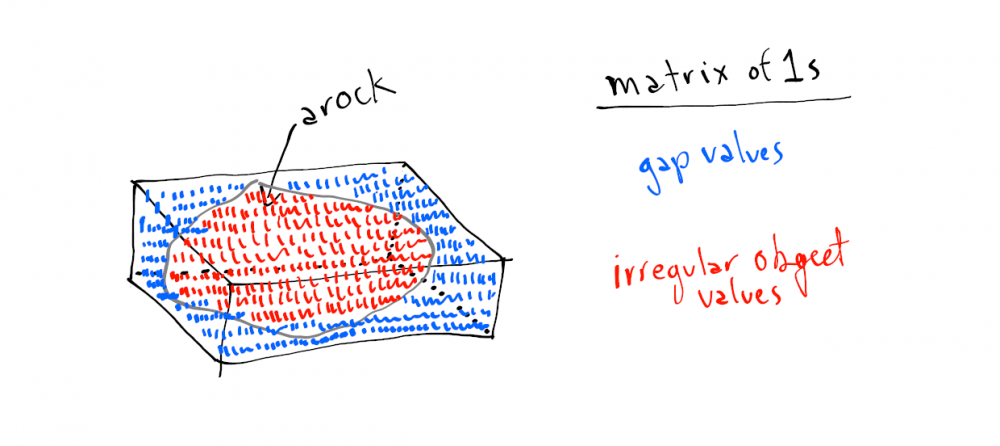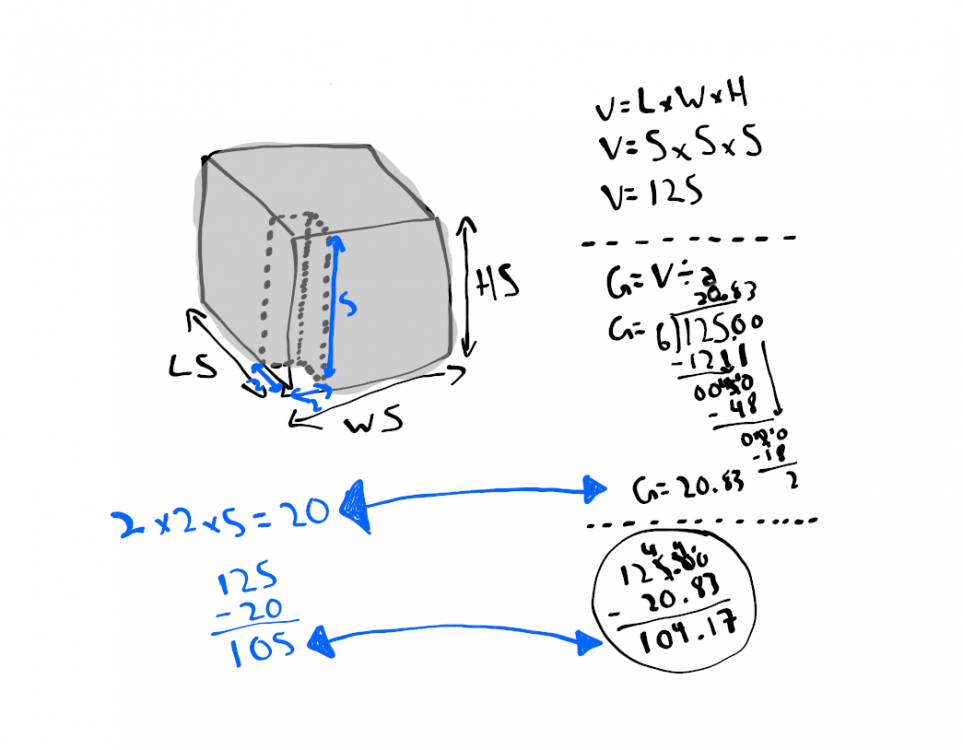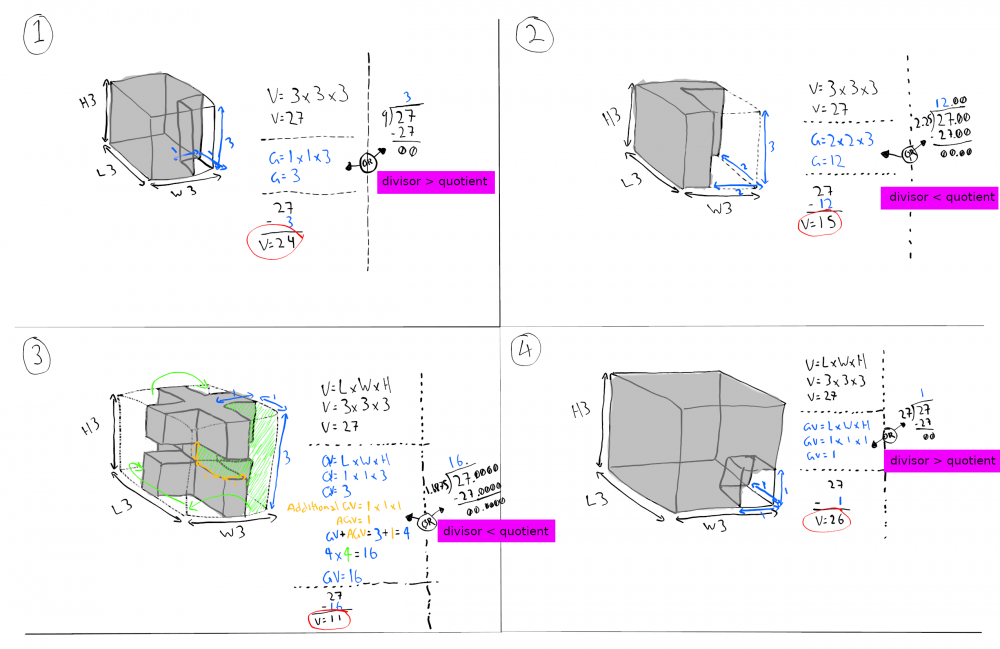# Volume Measurement

## Recommended Posts

Water Displacement Method

This web-page says to use water displacement method to do so:

Another Way?

But, is there a way to measure an irregular object without having to use the water displacement method for practical use with everyday objects?

Gap Value

The interesting thing about the formula for square or rectangled volume is that if used to measure irregular shaped objects, it would already contain gap information or gap values. The next step would be to find out how many amount gap number is then use total volume to subtract it to obtain an accurate volume for irregular object being measured. But the thing is I don't know how to obtain the amount of gap value number.

Why Gap Value?

Since the math is used as tool so the value number for every space occupied is 1 from my perspective. The reason for this is because I see an irregular object, when measured with volume formula LxWxH, as being a local matrix of 1s occupying every dimensional space as being uniform. The gap would be the space occupied in the measured volume.

Picture attached to show what I'm talking about.##### Share on other sites

What you talk about seems more like a method for calculation to me than an actual measurement. You can indeed calculate the volume of a body by taking a surrounding volume and then subtracting the parts of the surrounding volume that does not belong to the body (in your language: the volume filled with gap values). This may in some cases be an efficient method, e.g. for a block of stone with a cylindrical hole. In the general case, if you have a generic way to calculate the amount of gaps then you could probably use that method to calculate the volume of the body in the first place. This, as you already mentioned, is the tricky part.

The most generic way to approach this is dividing a volume in tiny blocks of simply-calculated sub-volumes and use these blocks to approximate the total volume from the sum of these blocks. For example, for your stone you could use small cubes and use laser scans to determine if a cube contains rock or not. If you make these blocks finer and add a few Greek letters, then you get what in Mathematics is called "integration", which is the theoretical basis for such measurements. In many semi-geometric structures you can get good results by gluing together and substracting known geometric structures (as in the case with the block with a cylindrical hole).

##### Share on other sites

On 2/15/2021 at 6:24 PM, timo said:

What you talk about seems more like a method for calculation to me than an actual measurement. You can indeed calculate the volume of a body by taking a surrounding volume and then subtracting the parts of the surrounding volume that does not belong to the body (in your language: the volume filled with gap values). This may in some cases be an efficient method, e.g. for a block of stone with a cylindrical hole. In the general case, if you have a generic way to calculate the amount of gaps then you could probably use that method to calculate the volume of the body in the first place. This, as you already mentioned, is the tricky part.

The most generic way to approach this is dividing a volume in tiny blocks of simply-calculated sub-volumes and use these blocks to approximate the total volume from the sum of these blocks. For example, for your stone you could use small cubes and use laser scans to determine if a cube contains rock or not. If you make these blocks finer and add a few Greek letters, then you get what in Mathematics is called "integration", which is the theoretical basis for such measurements. In many semi-geometric structures you can get good results by gluing together and substracting known geometric structures (as in the case with the block with a cylindrical hole).

I think I might found a solution for the tricky part, the amount of gap values.

Solution

Step 1: Total Volume, V = L x W x H.

Step 2: Division, Gap (quotient) = dividend (volume) / divisor (guess). Divide the volume with an educational guessed number of divisor that will get the dividend down to zero the most direct or shortcut way. The quotient answer is the amount number of gap value.

Step 3: Subtraction, V - Gap. Subtract the volume with the amount number of gap value to obtain an approximately close or even accurate number of volume number or information for an irregular object being measured and calculated.

How did I know

I was writing down math stuff then used imaginative answer that yielded from measuring imaginative semi-irregular objects (for easier measurement and calculation) then compared it against the division solution with calculator, either answer seems to approximately close to right however except for decimal and maybe rounding issues in some cases.

Uncertainty

I am a bit uncertain if this is a correct solution or not. Because of decimal and rounding maybe based on comparisons between my perform math and used calculator. (See attached picture to show what I'm talking about.)##### Share on other sites

Updated: The more correct divisor for the shown and attached picture is actually 6.25 , however I still am not sure or certain as how to obtain that divisor number. The guessing process with divisor is not exactly helpful. Another interesting thing to note; the quotient answer as per repeated pattern from some examples I produced seems to be always in number greater than divisor.. this maybe could be a clue to finding out how to obtain a correct divisor at first place.

##### Share on other sites

Division Method to Obtain Amount of Gap Value Information

Seems the division method is there to use. But it involves guessing which precisely and potential divisor or quotient number it is. Or a calculator is there to use to speed up the guess process or alternatively can develop a table with division of numbers ranging from 1.00 to how many maximum number specified on left (potential divisor or quotient) and upper (potential quotient or divisor), also with dividends (total volume) in contents (middle). However, this would involve an insanely large workload with Calc or Excel.. but the result would speed up the searching process for finding and identifying the divisor on order to obtain a gap value (quotient) then subtract volume with it to get a final volume amount for any irregular object.

Divisor and Quotient Issue

The issue with current process (including the division method and guessing) as per shown picture and previously updated post is that even if divisor is found (e.g. 6.25), and the quotient answer is 20 gap values or conversely (eg. 6.25 is the quotient and 20 is the divisor).. how to know which one is the correct divisor and quotient according to any situation? In a situation with the shown picture, it already supplied with additional information (e.g. predefined measurements - blue coloured). Seems more information is required to supply in order to complete the volume math measurement and calculation.

Reason for Gap Values

This could be a possible practical and efficient measurement & calculation process. Also, to obtain an accurate volume number of any irregular object based on an finite amount of information (eg. just LxWxH). When an irregular object is measured with the simple volume measurement, it would already contain gap information. If a better solution is found regarding the amount of gap information and division method, it could be useful somehow.

##### Share on other sites

Work in Progress

I have written down imaginary measurements and calculations based on my depicted semi-irregular objects; there are four examples of that. All of it is on attached picture to this post. It is a part of my work in progress regarding finding or identifying a pattern in divisor, quotient, and a possible relation between both - for to better a method to obtain a gap value, number, or information from a limited volume information.

Pattern in Divisor, Quotient, and Relation Between Both

The pattern seems to show up is: more gap values means divisor is less than quotient while less gap values means divisor is greater than quotient. A possible ratio or proportion could be involved in that, but I'm not sure. Also, seems a clue or guessing process with divisor could be that finding out a divisor number that will get dividend down to zero the most direct way but I'm not sure about that as well.

Example 1 to 4

Each depicting a simple volume measurement of semi-irregular object with various volume take-up (gray coloured) coupled with gap values already contained (blue coloured). And calculations (mostly black coloured) as well. Each example also has a comparison indicator (pink coloured - less or greater) for divisor and quotient.

The Point

If in any situation or event, a measurement of gap values is not doable, then what a reliable method to measure and calculate a volume of both everyday semi-irregular and irregular objects? That is what I'm trying to find out. Because presently measurement and calculation performed by myself for semi-irregular and irregular objects without gap measurement information, seems to be doable by division but with unpredictable result (e.g. guessing which precisely divisor and quotient it is). Seems additional visual information and / or other measurement methods will have to do for now.##### Share on other sites

No Method?

Seems there is no way to obtain an accurate divisor or quotient (two missing variables) in a case of no measurement information on gap value number(s). Though, the division is there to be used.. but that would be unreliable and unpredictable (e.g. guessing) especially without two variables and gap value number(s). Or optionally can develop a spreadsheet with all possible numbers in division and decimals pertaining to the volume, then can try look up some approximately close to correct answer to identify divisor and quotient.. but still.

Interesting Things to Note

- The 1-4 examples picture I posted, the final ratio when added up together per (ex1 - 24:3, ex2 - 15:12, ex3 - 11:16, and 26:1) is always pattern of nine or 9, I'm a bit not sure what it is referring to.. the 9 blocks of 27, or something else?

- If pattern of 9 x 3, we get 27 which is total volume of shown examples. This seems to show that the process is correct.

Wrapping It Up

I know when to stop, as I have been at this volume rabbit hole for around 1 week now. I guess I wanted an easy way to measure and obtain accurate volume number without having to use other ways of measuring and calculating - for any object.

## Create an account

Register a new account